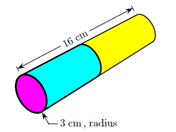# Problem: A cylinder, 16 cm long and 3 cm in radius, is made of two different metals bonded end-to-end to make a single bar. The densities are 4.4 g/cm3 and 6.3 g/cm3. What length of the lighter-density part of the bar is needed if the total mass is 2377 g? 1. 9.92098 2. 13.1373 3. 7.52775 4. 12.5493 5. 11.7805 6. 10.4417 7. 9.5286 8. 9.69549 9. 8.8057 10. 10.0839

###### FREE Expert Solution
99% (146 ratings)
###### Problem Details

A cylinder, 16 cm long and 3 cm in radius, is made of two different metals bonded end-to-end to make a single bar. The densities are 4.4 g/cm3 and 6.3 g/cm3. What length of the lighter-density part of the bar is needed if the total mass is 2377 g?

1. 9.92098

2. 13.1373

3. 7.52775

4. 12.5493

5. 11.7805

6. 10.4417

7. 9.5286

8. 9.69549

9. 8.8057

10. 10.0839Frequently Asked Questions

What scientific concept do you need to know in order to solve this problem?

Our tutors have indicated that to solve this problem you will need to apply the Density concept. You can view video lessons to learn Density. Or if you need more Density practice, you can also practice Density practice problems.

How long does this problem take to solve?

Our expert Physics tutor, Jeffery took 6 minutes undefined to solve this problem. You can follow their steps in the video explanation above.

What professor is this problem relevant for?

Based on our data, we think this problem is relevant for Professor Erskine's class at TEXAS.